Methods and formulas for 1 Variance

Select the method or formula of your choice.

Standard deviation (StDev)

The standard deviation is the most common measure of dispersion, or how spread out the data are about the mean. The sample standard deviation is equal to the square root of the sample variance.

If the column contains x1, x2,..., xN, with mean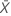, then the standard deviation is given by:

Notation

TermDescription
xithe ith observation in your samplethe sample mean
Sthe sample standard deviation
nsample size

Variance

The variance measures how spread out the data are about their mean. The variance is equal to the standard deviation squared.

Notation

TermDescription
xiith observationmean of the observations
Nnumber of nonmissing observations

Confidence intervals and bounds for the chi-square method

Use this method when your data are normally distributed. This method is inaccurate for nonnormal data, even when the sample size is very large.

Confidence intervals

A 100(1 - α)% confidence interval for the population standard deviation is given by:
A 100(1 - α)% confidence interval for the population variance is given by:

Confidence bounds

When you specify a one-sided test, Minitab calculates a one-sided 100(1–α)% confidence bound, according to the direction of the alternative hypothesis.

• If you specify a "greater than" alternative hypothesis, a 100(1–α)% lower bound for the population standard deviation is given by:

A 100(1–α)% lower bound for the population variance is given by:

• If you specify a "less than" alternative hypothesis, a 100(1–α)% upper bound for the population standard deviation is given by:

A 100(1–α)% upper bound for the population variance is given by:

Notation

TermDescription
αalpha-level for the 100(1 – α)% confidence interval
nsample size
S2sample variance
Χ2(p)the upper 100pth percentile point on a chi-square distribution with (n – 1) degrees of freedom
σtrue value of the population standard deviation
σ2true value of the population variance

Confidence intervals and bounds for the Bonett method

Use this method for any continuous data (normal or nonnormal). 1

Confidence interval

A 100(1-α)% confidence interval for the population standard deviation is given by:
A 100(1-α)% confidence interval for the population variance is given by:

Confidence bounds

When you specify a one-sided test, Minitab calculates a one-sided 100(1–α)% confidence bound, according to the direction of the alternative hypothesis.

• If you specify a "greater than" alternative hypothesis, a 100(1–α)% lower bound for the population standard deviation is given by:
An approximate 100(1- a)% lower bound for the population variance is given by:
• If you specify a "less than" alternative hypothesis, an approximate 100(1 – α)% upper bound for the population standard deviation is given by:
An approximate 100(1- a)% upper bound for the population variance is given by:

Notation

TermDescription
α 1 – confidence level / 100
cα/2 n / (nzα/2)
cα n / (nzα )
s2 observed value of the sample variance
zα/2 inverse cumulative probability of the standard normal distribution at 1 – α/2. If n is less than or equal to zα/2, Minitab does not calculate Bonett confidence intervals.
zα inverse cumulative probability of the standard normal distribution at 1 – α. If n is less than or equal to zα , Minitab does not calculate Bonett confidence intervals.
se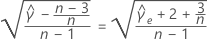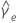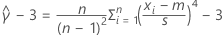= estimated excess kurtosis
m trimmed mean with trim proportion equal to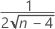; m = sample mean when n is less than or equal to 5
σ true value of the population standard deviation
σ2 true value of the population variance

Hypothesis test for the chi-square method

Use this method when your data are normally distributed. The method is inaccurate for nonnormal data, even when the sample size is very large.

Formula

The hypothesis test uses the following p-value equations for the respective alternative hypotheses:

H1: σ2 > σ02: p-value = P(Χ2x2)

H1: σ2 < σ02: p-value = P(Χ2x2)

H1: σ2σ02: p-value = 2 × min{P(Χ2x2), P(Χ2x2)}

Notation

TermDescription
σ2true value of the population variance
σ02hypothesized value of the population variance
Χ2follows a chi-square distribution with (n – 1) degrees of freedom when σ2 = σ02
x2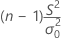TermDescription
S2observed value of the sample variance
nsample size

Hypothesis test for the Bonett method

Use this method for any continuous data (normal or nonnormal).

Formula

The Bonett procedure is not associated with a test statistic. However, Minitab uses the rejection regions defined by the confidence limits to calculate a p-value.

For a two-sided hypothesis, the p-value is given by:

p = 2 × min(αL, αU)

• For a one-sided "less than" alternative hypothesis, the p-value is calculated as αU after replacing α/2 with α in the notation.
• For the one-sided "greater than" alternative hypothesis, the p-value is calculated as αL after replacing α/2 with α in the notation.

Notation

TermDescription
σ02hypothesized variance
αLsmallest solution, α, of the equation
αUsmallest solution, α, of the equation
cα/2n / (nzα/2)
α1 – confidence level / 100
s2observed value of the sample variance
zα/2inverse cumulative probability of the standard normal distribution at 1 – α/2. If n is less than or equal to zα/2, Minitab does not calculate Bonett confidence intervals.
seTermDescription= estimated excess kurtosis
mtrimmed mean with trim proportion equal to; m = 0 when n is less than or equal to 5
1 D.G. Bonett (2006). "Approximate confidence interval for standard deviation of nonnormal distributions" , Computational Statistics & Data Analysis, 50, 775-782.
By using this site you agree to the use of cookies for analytics and personalized content.  Read our policy